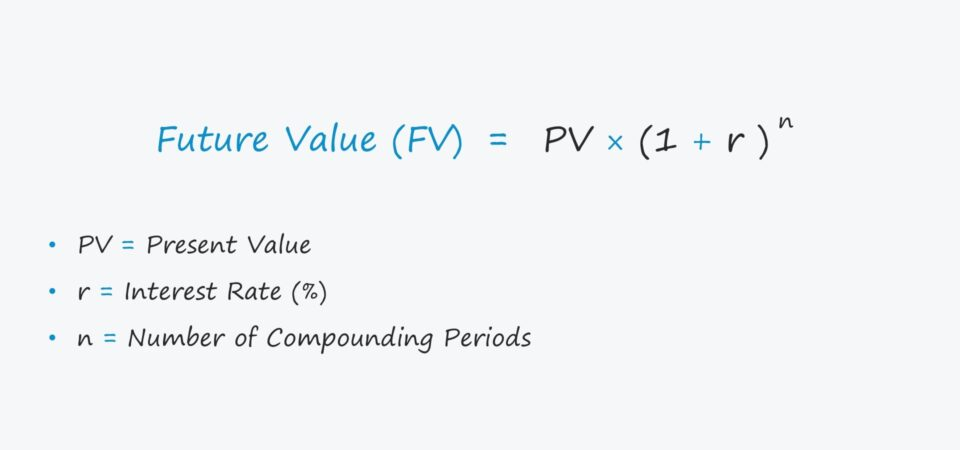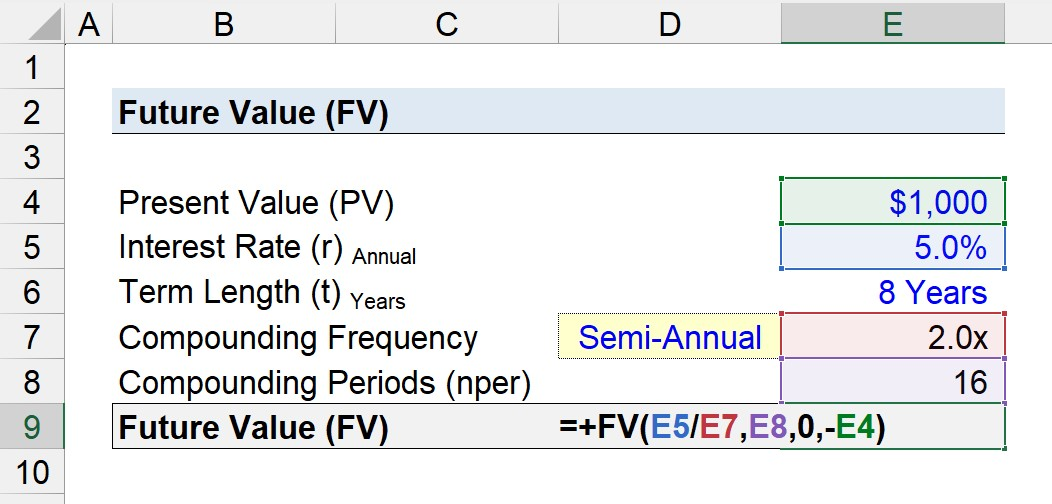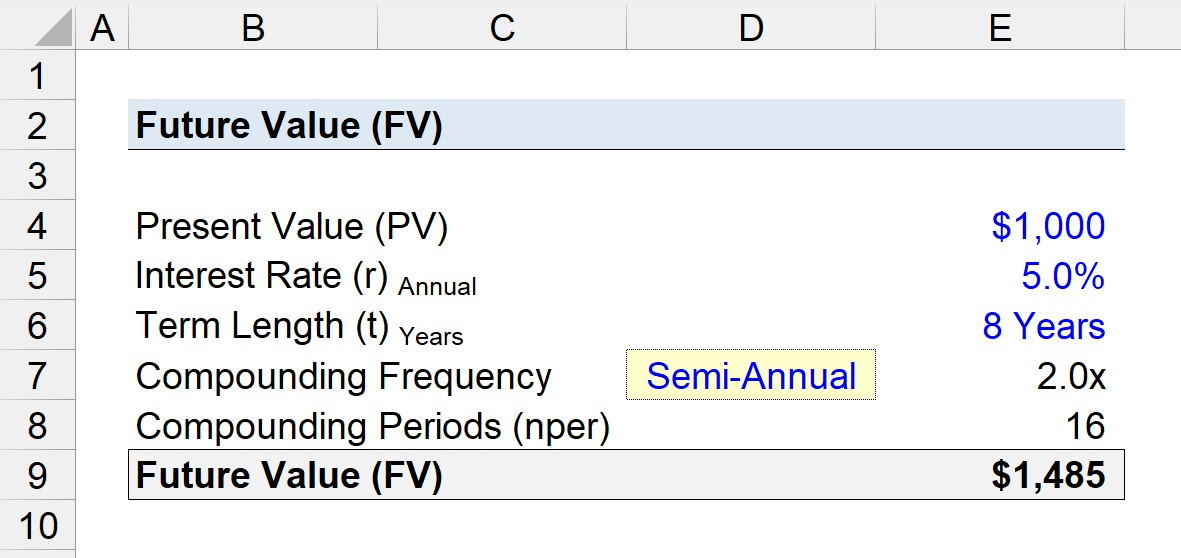Welcome to Wall Street Prep! Use code at checkout for 15% off.# Future Value (FV)

Guide to Understanding Future Value (FV)## How to Calculate Future Value (FV)?

The future value (FV) is a fundamental concept to corporate finance, whether it be for determining the valuation of a potential investment or projecting cash flows to support capital budgeting decisions.

For investors and corporations alike, the future value is calculated to estimate the value of an investment at a later date to guide decision-making.

The calculated future value is a function of the interest rate assumption – i.e. the rate of return earned on the original amount of capital invested, or the present value (PV).

The present value (PV) is defined as the initial investment amount, whereas the future value represents the ending amount, with the original amount as well as any accumulated interest.

The “time value of money” states that a dollar today is worth more than a dollar tomorrow, so future cash flows must be discounted back to the present date to be comparable to present values.

There are two types of interest: 1) simple interest and 2) compound interest.

1. Simple Interest: The amount of interest earned is calculated off the original principal (or deposit) amount, which remains constant throughout the investment horizon.
2. Compound Interest: The incremental amount of interest earned is calculated off the original principal amount (or deposit) and the accrued interest to date, i.e. “interest on interest”.

## Future Value Formula (FV)

The formula used to calculate the future value is shown below.

Future Value (FV) = PV × (1 + r) ^ n

Where:

• PV = Present Value
• r = % Interest Rate
• n = Number of Compounding Periods

## How Compounding Frequency Impacts Future Value?

The number of compounding periods is equal to the term length in years multiplied by the compounding frequency.

The more compounding periods there are, the greater the future value is going to be.

• Annual Compounding = 1x
• Semi-Annual Compounding = 2x
• Quarterly Compounding = 4x
• Monthly Compounding = 12x
• Daily Compounding = 365x

For example, if you decided to invest \$100.00 at an interest rate of 10% – assuming a compounding frequency of 1 – the investment should be worth \$110 by the end of one year.

• Future Value (FV) = \$100 × (1 + 10%) ^ 1 = \$110.00

However, if the interest compounds semi-annually, the investment is worth \$121 instead.

• Future Value (FV) = \$100 × (1 + 10 ÷ 2%) ^ 2 = \$110.25

## Future Value Calculator (FV)

We’ll now move to a modeling exercise, which you can access by filling out the form below.Submitting...

## 1. FV of Bond Calculation in Excel

Suppose a corporate bond has a present value (PV) of \$1,000 with a stated annual interest rate of 5.0%, which compounds on a semi-annual basis.

If we assume that the term length is 8 years – the following are the inputs to calculate the future value of the deposit.

• Present Value (PV) = \$1,000
• Annual Interest Rate (r) = 5.0%
• Term Length (t) = 8 Years
• Compounding Frequency = Semi-Annual (2x)

Since the number of compounding periods is equal to the term length (8 years) multiplied by the compounding frequency (2x), the number of compounding periods is 16.

• Number of Compounding Periods (nper) = 8 Years × 2 = 16

## 2. Future Value Calculation Example (FV)

The “FV” function in Excel can be used to determine the value of the \$1,000 bond after an eight-year time frame.

= FV(rate, nper, pmt, pv)

Note, a negative sign must be placed in front of the present value input for the Excel function to work as intended.## 3. FV Calculation Example in Excel

If we enter our assumptions into the Excel formula, we arrive at a future value (FV) of \$1,485.

=FV(5.0% ÷ 2, 16, 0, –\$1,000)

So the bond has increased from \$1,000 to \$1,485 after eight years, given the annual interest rate of 5.0% compounded on a semi-annual basis.

The more frequently that the deposit is compounded, the greater the amount of interest earned, which we can confirm by adjusting the compounding frequency.

• Annual Compounding = \$1,477
• Semi-Annual Compounding = \$1,485
• Quarterly Compounding = \$1,488
• Monthly Compounding = \$1,491

In conclusion, the implied future value (FV) of the bond increases with a higher frequency of compounding.Step-by-Step Online Course

### Everything You Need To Master Financial Modeling

Enroll in The Premium Package: Learn Financial Statement Modeling, DCF, M&A, LBO and Comps. The same training program used at top investment banks.Inline FeedbacksLearn Financial Modeling Online

Everything you need to master financial and valuation modeling: 3-Statement Modeling, DCF, Comps, M&A and LBO.

X

The Wall Street Prep Quicklesson Series

7 Free Financial Modeling Lessons

Get instant access to video lessons taught by experienced investment bankers. Learn financial statement modeling, DCF, M&A, LBO, Comps and Excel shortcuts.